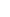8. WHY DOES ALICE LAW END THE RELATIVITY THEORY?I guess you are really curious about the answer to this question, so I would like to give an answer in order not to keep you waiting.The reason Theory of Relativity emerged was the assumption that the way light behaves is not in line with The Rule of Velocity Addition. In sum, it was thought that speed of light was constant relative to all reference systems and Theory of Relativity arose from this base.Getting back to our example consisting of two boxes, lets handle the topic here again for Theory of Relativity. Both the frames were sending each other signals when there was d0 distance between them.Taking Theory of Relativity into consideration, relative to the reference systems of Frame A and Frame B, the speeds of INCOMING and OUTGOING signals must be constant, that is, c. The mathematics of The Theory of Relativity has been shaped on this path that represents this logic. The results that this mathematics indicates emerged as the logical results of The Theory of Relativity. You have definitely heard of some of these results such as slowing down of moving clocks, shortening of the lengths of objects in the direction of the movement, big celestial bodies bending nearby space, etc.When we look through the window of Alice Law, the situation is completely different. We came up with the result of c for the INCOMING signal and (c+v) or (c-v) for OUTGOING signal. Alice Law is defined by (c+v) (c-v) mathematics and this mathematics basically shows that, although the behavior of light is a bit different, it is in line with the Velocity Addition Rule. Similarly, (c+v) (c-v) mathematics indicates results that belong to it. These are the results of Alice Law and most of these are explained in this book.For the solution to the same problem, it is necessary that the mathematical methods that Alice Law and Theory of Relativity use are different and that different answers are obtained; and naturally, this means that only one of these mathematics are correct. The correct one will survive and the wrong one will be eliminated; there is no way both can live. When one of these mathematics is eliminated, all of its logical and mathematical results will disappear along with it. It is that simple.Alice Law eliminated Theory of Relativity by showing that it is wrong. While Alice Law placed itself in the space Theory of Relativity left, it put all the results that its own mathematics brought along with it in their appropriate places.